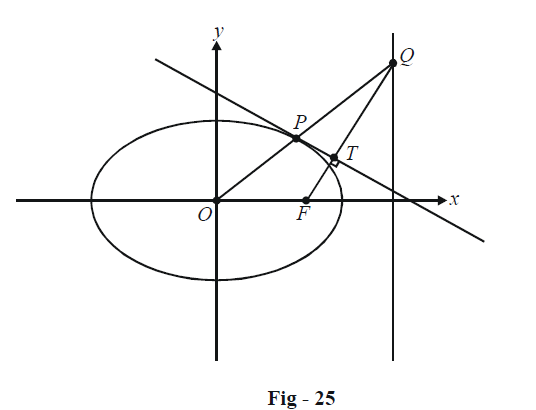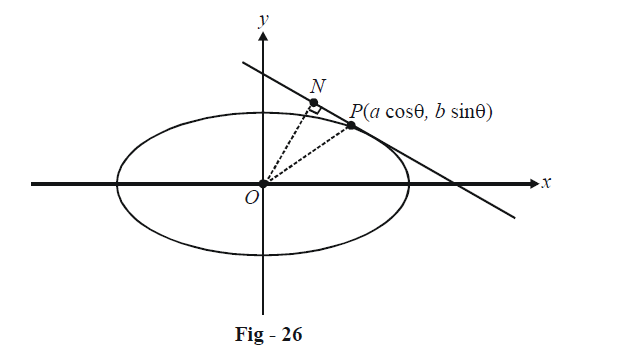# Examples On Tangents To Ellipses Set-4

Go back to  'Ellipse'

Example - 22

Prove that in any ellipse, the perpendicular from a focus upon any tangent and the line joining the centre of the ellipse to point of contact meet on the corresponding directrix.

Solution: Let the ellipse be \begin{align}\frac{{{x^2}}}{{{a^2}}} + \frac{{{y^2}}}{{{b^2}}} = 1\end{align}  and let a tangent be drawn to it at an arbitrary point $$P(a\cos \theta ,\,b\sin \theta )$$ as shown :We need to show that the perpendicular from F onto this tangent, i.e., FT, and the line joining the centre to the point of contact, i.e. OP intersect on the corresponding directrix; in other words, we need to show that the x-coordinate of Q as in the figure above is \begin{align}x = \frac{a}{e}.\end{align}

The equation of the tangent at P is $$\frac{x}{a}\cos \theta + \frac{y}{b}\sin \theta = 1.$$

The slope of this tangent is $${m_T} = \frac{{ - b}}{a}\cot \theta$$

Therefore, the slope of FT is

${m_{FT}} = \frac{a}{b}\tan \theta$

The equation of FT is

$FT: y = \left( {\frac{a}{b}\tan \theta } \right)(x - ae)\quad\quad\quad...\left( 1 \right)$

The equation of OP is simply

$OP:y = \left( {\frac{b}{a}\tan \theta } \right)x \quad\quad\quad...\left( 2 \right)$

Comparing (1) and (2) gives $$x = a/e,$$ which proves the stated assertion.

Example - 23

Find the coordinates of all the points on the ellipse \begin{align}\frac{{{x^2}}}{{{a^2}}} + \frac{{{y^2}}}{{{b^2}}} = 1\end{align} for which the area of $$\Delta PON$$ is the maximum where O is the origin and N is the foot of the perpendicular from O to the tangent at P.

Solution: We can assume the point P to be $$(a\cos \theta ,\,b\sin \theta )$$ so that the tangent at P has the equation

$\frac{x}{a}\cos \theta + \frac{y}{b}\sin \theta = 1\quad\quad\quad...\left( 1 \right)$To evaluate the area of $$\Delta PON,$$ we first need the coordinates of the point N. The equation of ON is

\begin{align}&y - 0 = \frac{a}{b}\tan \theta (x - 0)\\ &\Rightarrow\quad ax\sin \theta - by\cos \theta = 0\qquad\qquad...\left( 2 \right)\end{align}

The intersection of (1) and (2) gives us the coordinates of N as

$N \equiv \left( {\frac{{a{b^2}\cos \theta }}{{{a^2}{{\sin }^2}\theta + {b^2}{{\cos }^2}\theta }},\,\frac{{{a^2}b\sin \theta }}{{{a^2}{{\sin }^2}\theta + {b^2}{{\cos }^2}\theta }}} \right)$

The length PN can now be evaluated using the distance formula :

$PN = \frac{{({a^2} - {b^2})\sin \theta \cos \theta }}{{\sqrt {{a^2}{{\sin }^2}\theta + {b^2}{{\cos }^2}\theta } }}$

The length ON is simply the perpendicular distance of O from the tangent at P given by (1) :

$ON = \frac{{ab}}{{\sqrt {{a^2}{{\sin }^2}\theta + {b^2}{{\cos }^2}\theta } }}$

Thus, the area of $$\Delta OPN$$ is

\begin{align}&\Delta = \frac{1}{2} \times PN \times ON\\&\quad{\rm{ }} = \frac{{ab({a^2} - {b^2})}}{2}\frac{{\sin \theta \cos \theta }}{{{a^2}{{\sin }^2}\theta + {b^2}{{\cos }^2}\theta }}\\&\quad{\rm{ }} = \frac{{{a^2} - {b^2}}}{2}\frac{1}{{\frac{a}{b}\tan \theta + \frac{b}{a}\cot \theta }}\end{align}

The expression $$\frac{a}{b}\tan \theta + \frac{b}{a}\cot \theta$$ is of the form $$y + \frac{1}{y}$$ whose minimum magnitude is 2, when

\begin{align}&\frac{a}{b}\tan \theta = \pm 1\\ &\Rightarrow \quad\tan \theta = \pm \frac{b}{a}\\ & \Rightarrow\quad \sin \theta = \pm \frac{b}{{\sqrt {{a^2} + {b^2}} }},\,\cos \theta = \pm \frac{a}{{\sqrt {{a^2} + {b^2}} }}\end{align}

When this minimum is achieved, $$\Delta$$ is maximum. Thus, the possible coordinates of P for which area $$(\Delta OPN)$$ is maximum are

$P \equiv \left( { \pm \frac{{{a^2}}}{{\sqrt {{a^2} + {b^2}} }},\,\, \pm \frac{{{b^2}}}{{\sqrt {{a^2} + {b^2}} }}} \right)$

As might have been expected from symmetry, there are four such possible points on the ellipse.